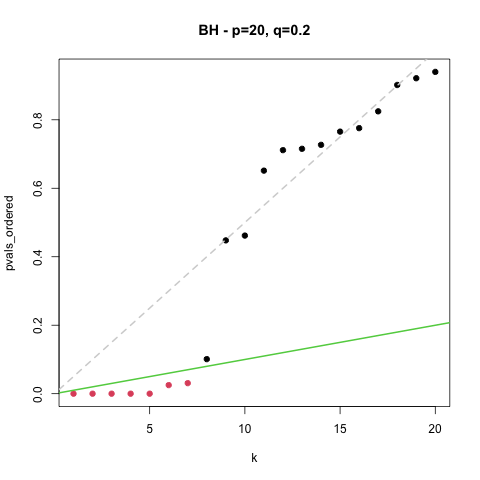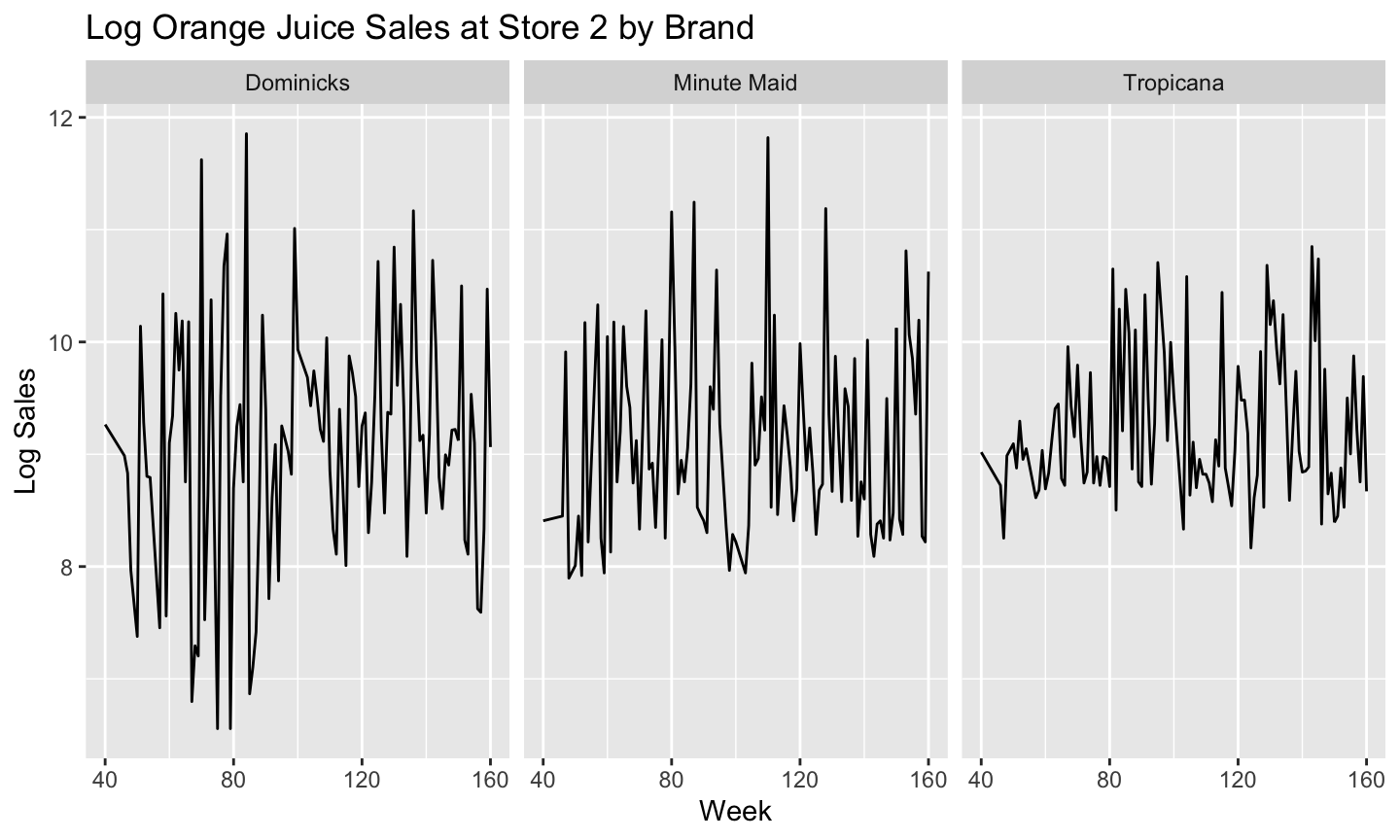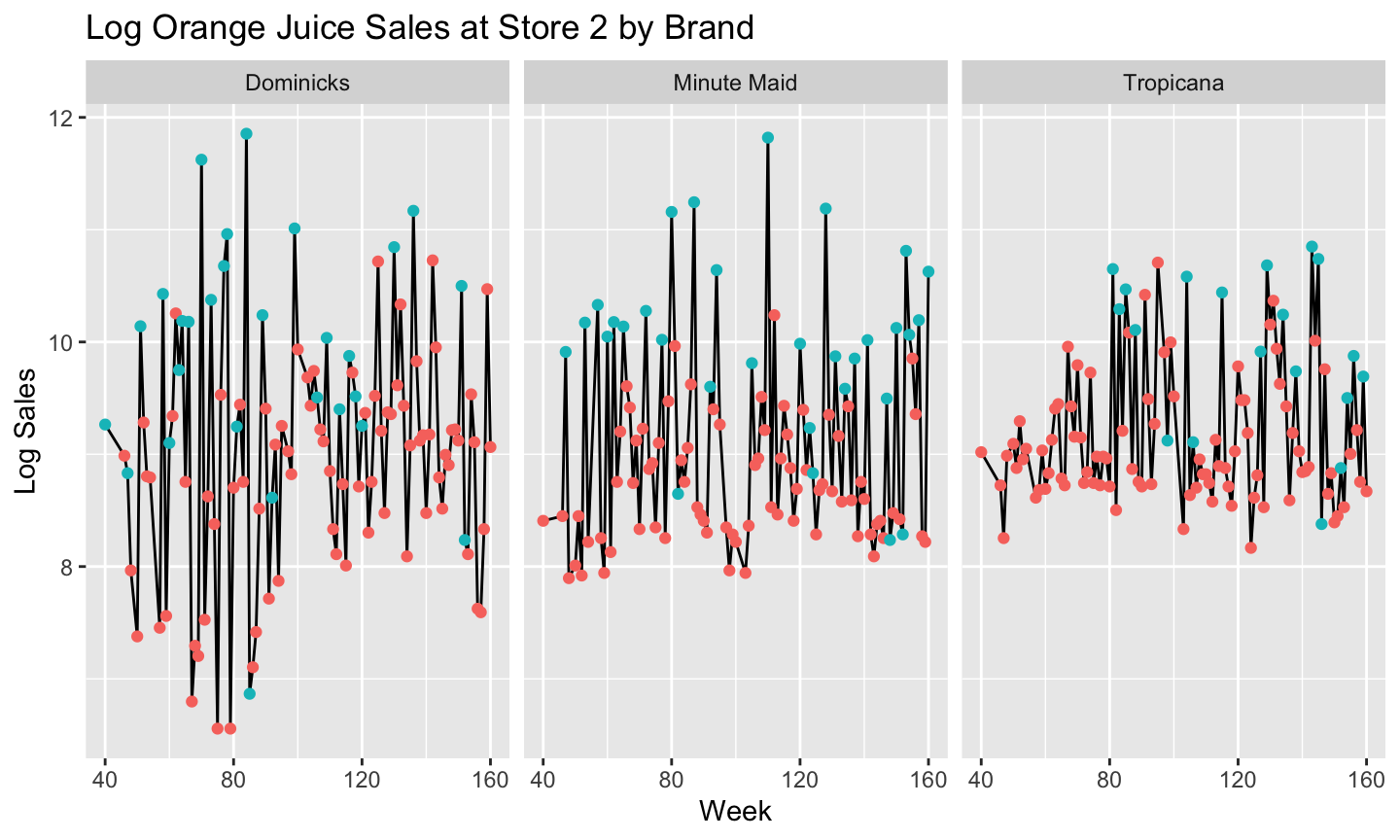April 6th, 2021

## Today’s Class

### Likely to spillover to Thursday

1. Quick Review
• FDR
• Loss
2. Regression Basics
• Single redux
• Multivariate
• Interactions
• Factors
3. Logistic Regression
4. Deviance
• Out-of-sample

## FDR RoundupWe started with the notion that a given $$\alpha$$, (pvalue cutoffs) can lead to a big FDR: $$\alpha \rightarrow q(\alpha)$$.

BH reverse that. They fix FDR, and find the relevant $$\alpha$$. The algorithm is the key to doing that. $$q \rightarrow \alpha^*(q)$$

## Loss

• Loss is a function both of our prediction and the true outcome
• More importantly, the driving feature of loss is our experience of making a certain error. Do we lose money? Time? Prestige?
• Our choice of procedure is driven by this loss.
• $$l_p(Y,\hat{Y}) = l_p(Y-\hat{Y}) = l_p(e) = \left( \frac1n \sum_{i=1}^n |e|^p\right)^{\frac{1}{p}}$$
• E.g. $$l_2(e) = \sqrt(\frac1n \sum_{i=1}^n e^2)$$.

## MotivationWhat is driving sales? Brand differences? Price changes? Ads?

## MotivationBlue points are based on ongoing promotional activity.
It looks like ads are important.

## Motivation

Fit a line for sales by brand controlling for promotional activity.

$log(Sales) \approx \alpha + \gamma Brand + \beta Ads$

$$\alpha+\gamma_b$$ are like our baseline sales. But we can bring in $$\beta$$ more sales with some promotional activity.

## Regression

• Regression through linear models
• Implementation in R
• Complications:
• Interaction
• Factors
• Logistic Regression
• Estimation: Maximum likelihood, Minimum Deviance

This should be mostly review, but perhaps with a different emphasis.

## Linear Models

Many problems involve a response or outcome (y),
And a bunch of covariates or predictors (x) to be used for regression.

A general tactic is to deal in averages and lines.

$E[y|x] = f(x'\beta)$

Where $$x = [1,x_1,x_2,x_3,...,x_p]$$ is our vector of covariates. (Our number of covariates is $$p$$ again)
$$\beta = [\beta_0,\beta_1,\beta_2,...,\beta_p]$$ are the corresponding coefficients.
The product $$x'\beta = \beta_0+\beta_1 x_1 + \beta_2 x_2+\cdots+\beta_p x_p$$.

For simplicity we denote $$x_0 = 1$$ to estimate intercepts